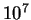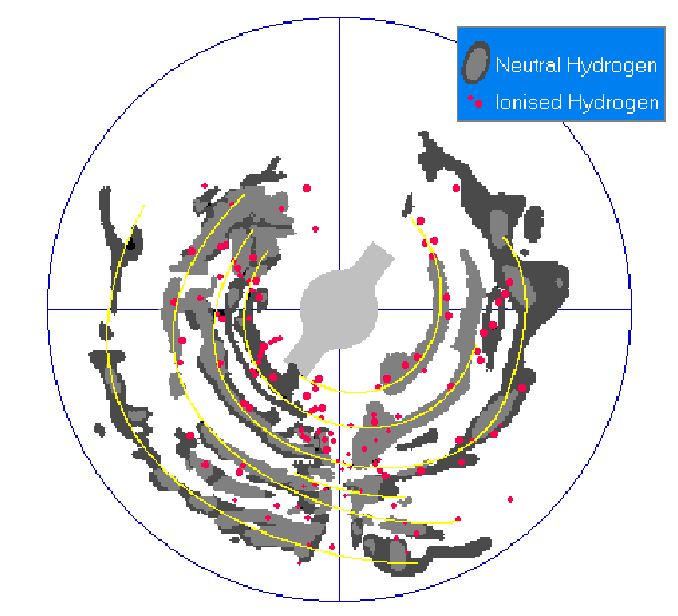Next: The Ionized Hydrogen Up: The Atomic Hydrogen Previous: The Atomic Hydrogen   Contents

### The 21 cm line

Neutral hydrogen is observable at radio frequencies, because its ground level term,, is split into two sublevels. This occurs because the electron spin and the proton spin may be either parallel or antiparallel. The electron angular momentum vector (J=L+S) can therefore combine in vector addition with the nuclear spin I to form a total angular momentum vector F=J+I. Two states F=1 (parallel spins) or F=0 (antiparallel spins) are possible and the second configuration has slightly lower energy than the first, giving two hyperfine levels. The statistical weights of the two levels are 3 and 1 respectively.
The energy separation is due to the interaction between the magnetic field produced by the moving electron and the magnetic moment of the nucleus. The energy difference between the two hyperfine levels is tiny; the transition between the two levels corresponds to a frequency of 1420.40575 MHz or a wavelength of 21.1049 cm. The transition probability is very small (), giving a radiative lifetime for the upper level ofyears. The lifetime of the state is so long that natural broadening is negligible, so that, when the line is optically thin, the line profile is determined entirely by kinetic temperature and velocity structure along the line of sight. The population balance between the two hyperfine levels is determined entirely by collisions, so that Boltzmann equilibrium pertains, and the relevant temperature is the spin temperature. In most cases however, the spin temperature can be taken as the kinetic temperature.
This emission shows a different Doppler shift depending on the zone of the galaxy from which it comes, combining these observations with the rotational curve of the Galaxy it is possible to reconstruct the three-dimensional structure of the Milky Way.Next: The Ionized Hydrogen Up: The Atomic Hydrogen Previous: The Atomic Hydrogen   Contents
Andrea Giuliani 2005-01-21